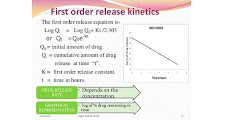## order of kinetics

FIRST ORDER KINETICS:
• Absorption, distribution, biotransformation and excretion processes are mostly occurring at rates proportional to the conc. of drug in the plasma
• A constant fraction of drug is absorbed, distributed, biotransformed and excreted per unit time
• These processes increase in rate with increase in conc. and decrease with falling conc.
• A constant fraction of the drug in the body is eliminated per unit time
• The rate of elimination is proportional to the amount of drug in the body
• The majority of drugs are eliminated in this way
• For drugs with first order kinetics the time required to achieve steady state levels can be predicted from Half life

Half life

• Elimination
1t1/2 50%
2t1/2
75%
3 t1/2
87.5%
4 t1/2 93.75%
5 t1/2 96.875
FIRST ORDER METABOLISM:
• A drug may be given in doses that produce blood concentrations less than the Km of the enyzme for the drug.
• v = Vmax [C]
• Km + [C]
• When Km >>> [C],
• then v = Vmax [C] & v σ [C]
• Km
• Metabolism of the drug is a first order process.
• Rate of elimination is directly proportional to drug concentration
• A constant fraction of the remaining drug is metabolized per unit time.
• Most drugs are given at concentrations smaller than the Km of the enzymes of their metabolism.
ZERO ORDER KINETICS:
• A constant amount of drug is eliminated per unit time
• Supply of enzymes are limited in the body
• So, with increasing dose a time will come when the supply of enzymes get saturated
• At this point no elimination occur
• Biotransformation or excretion remains constant at this point
• So, rate of processes or reaction is not proportional to the conc. or dose
• Processes showing such kinetics are known as – Rate limited or zero order or saturation kinetics
• Some times clinically called non-linear kinetics
• All enzyme mediated processes show this type of kinetics
• Passive diffusion like processes do not show this type of kinetics
• e.g. aspirin, ethanol, phenytoin ,propanolol, tolbutamide etc.

ZERO ORDER METABOLISM:
• A drug may be given in doses that produce blood concentrations greater than the Km of the enyzme for the drug.
v = Vmax [C]
K m + [C]v
When [C] >>> Km,
then v = Vmax [C] , and v = Vmax
[C]
• Metabolism of the drug is a zero order process.
• A constant amount of the remaining drug is metabolized per unit time.
• Phenytoin undergoes zero order metabolism at the doses given.
Drugs showing zero/pseudo-zero order kinetics :
• Phenytoin
• Theophylline
• Alcohol
• Tolbutamide
• Warfarin
• Salicylates (Aspirin)
• Propanolol

First Order Kinetics Zero Order Kinetics (Non linear Kinetics)

• Constant fraction of drug is eliminated per unit time
• Rate of elimination is proportional to plasma concentration
• Clearance remains constant
• Half life remains constant
• Most of the drugs follow first order kinetics

• Constant amount of the drug is eliminated per unit time.
• Rate of elimination is independent of plasma concentration
• Clearance is more at low concentrations and less at high,concentrations
• Half life is less at low concentrations and more at high concentrations
• Very few drugs follow pure zero order kinetics e.g. alcohol
• Any drug at high concentration (when metabolic or elimination pathway is saturated)
• may show zero order kinetics
Exam Question
• Zero order kinetics is independent of Plasma concentration
• Zero order kinetics occur in Phenytoin,Tolbutamide, Propranolol and Ethanol at high dose
• In first order kinetics a constant proportion of plasma concentration is eliminated
• In first order kinetics Rate of elimination is directly proportional to drug concentration
• Zero order kinetics is otherwise known as saturation kinetics
• In first order kinetics Clearance is constant
• Elimination after 4 half lives in first order Kinetics is 93%
• Elimination after 3 half lives in first order kinetics is 87.5%
• For drugs with first order kinetics the time required to achieve steady state levels can be predicted from Half life
• First order kinetics is Elimination of the drug is proportional to the serum concentration
• In Zero order kinetics Constant amount of drug eliminated per unit time
Don't Forget to Solve all the previous Year Question asked on order of kinetics